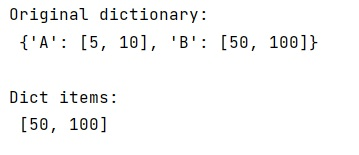# How to save dictionaries through numpy.save()?

Learn, how to save dictionaries through numpy.save() in Python?
Submitted by Pranit Sharma, on February 22, 2023

NumPy is an abbreviated form of Numerical Python. It is used for different types of scientific operations in python. Numpy is a vast library in python which is used for almost every kind of scientific or mathematical operation. It is itself an array which is a collection of various methods and functions for processing the arrays.

## Saving dictionaries through numpy.save()

Suppose that we are having a data set in the form of NumPy arrays and dictionaries. After the successful construction of this data set, we need to store them into files so that the files can be loaded into the memory quickly without reconstructing the data set from the beginning.

We can save and load the data set into the memory by using numpy.save() and Numpy.load(). Both of these methods work well with numpy arrays but in the case of a dictionary, it will load the data as a numpy array and not as a dict.

For this purpose, we need to use the dict.item() to retrieve the actual dict object first after saving and loading the data.

Let us understand with the help of an example,

## Python code to save dictionaries through numpy.save()

```# Import numpy
import numpy as np

# Creating a dict
d = {'A':[5,10], 'B':[50,100]}

# Display original dict
print("Original dictionary:\n",d,"\n")

# Saving the data
np.save("d.npy", d)

# Display dict items
print("Dict items:\n",data.item().get('B'))
```

Output: